### 2

2 is a prime number and is the only even prime number. = 1 x 2

The number two has many properties in mathematics. An integer is called even if it is divisible by 2. For integers written in a numeral system based on an even number, such as decimal and hexadecimal, divisibility by 2 is easily tested by merely looking at the last digit.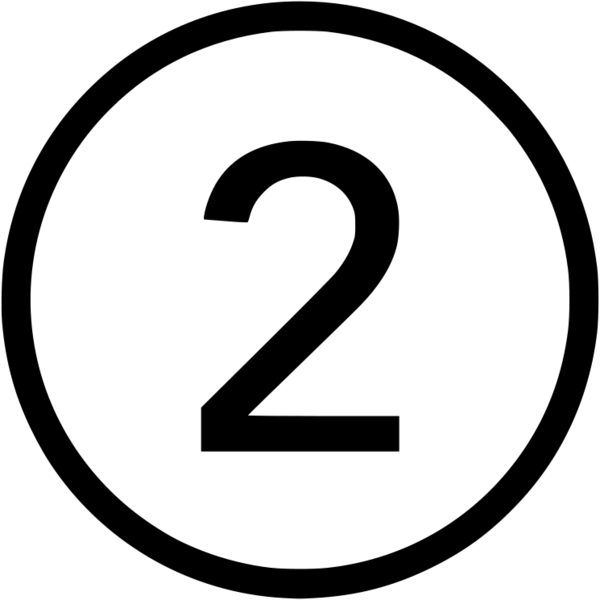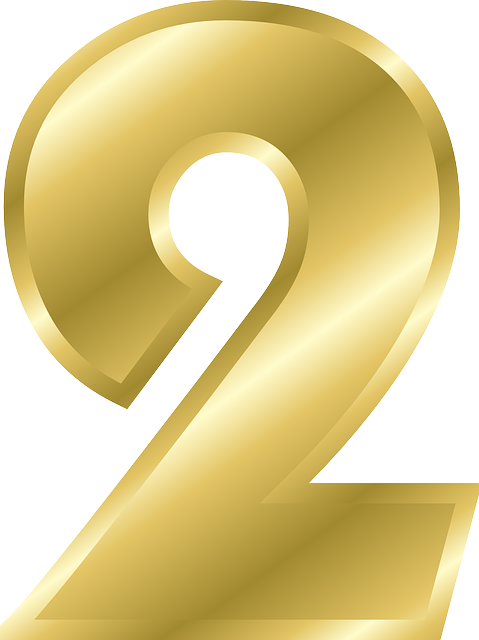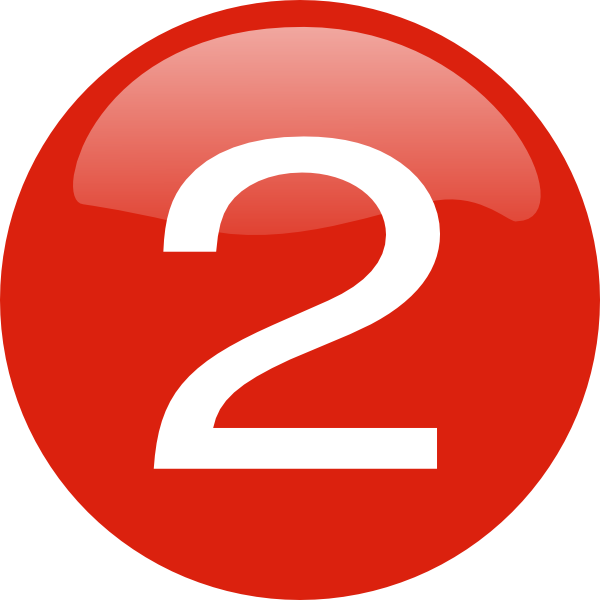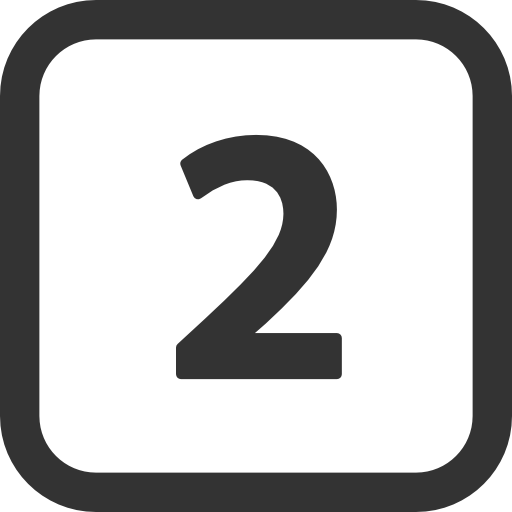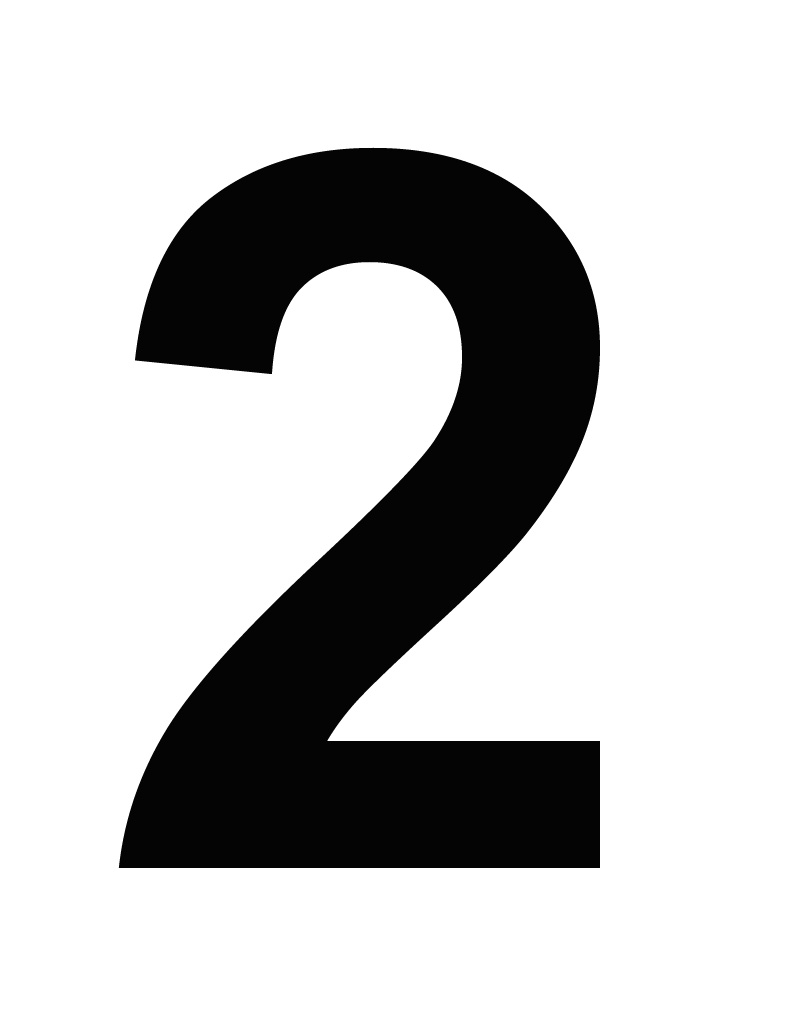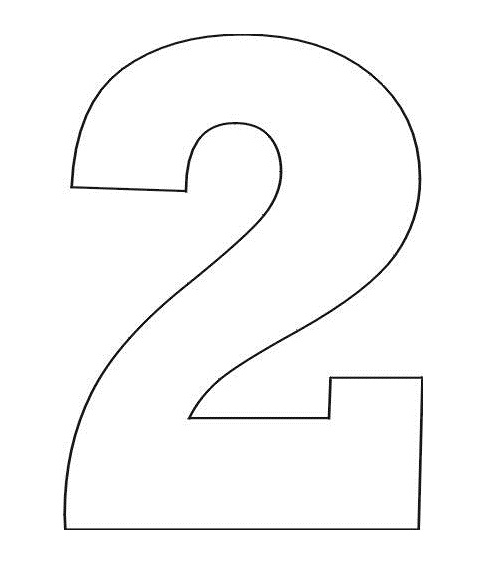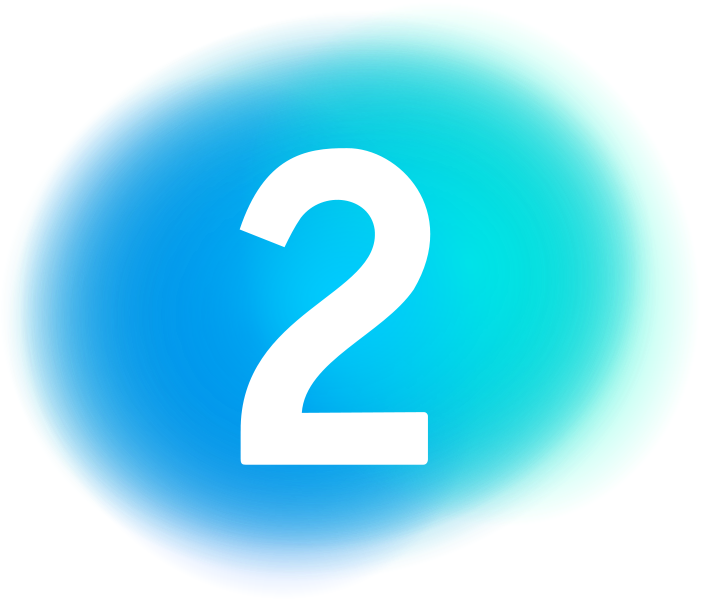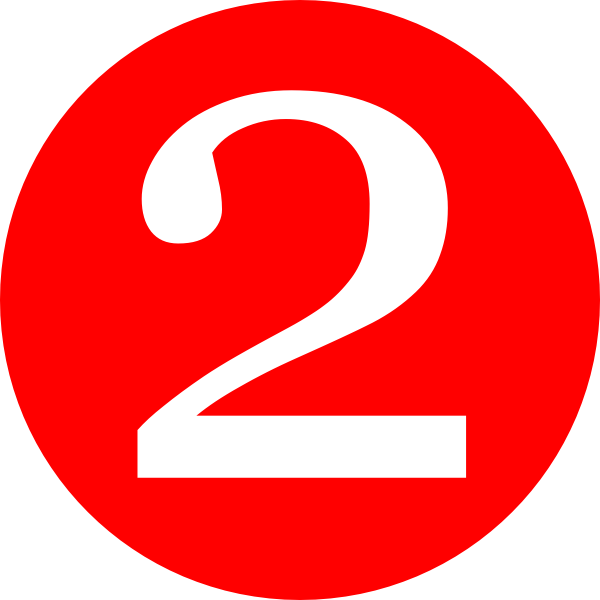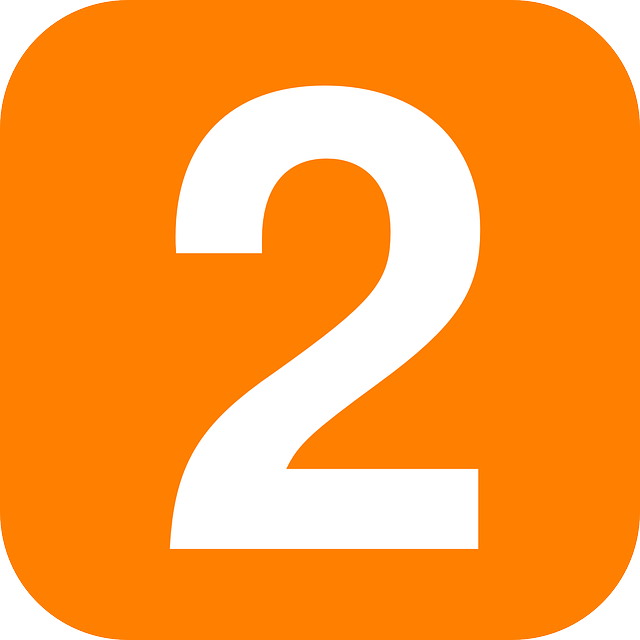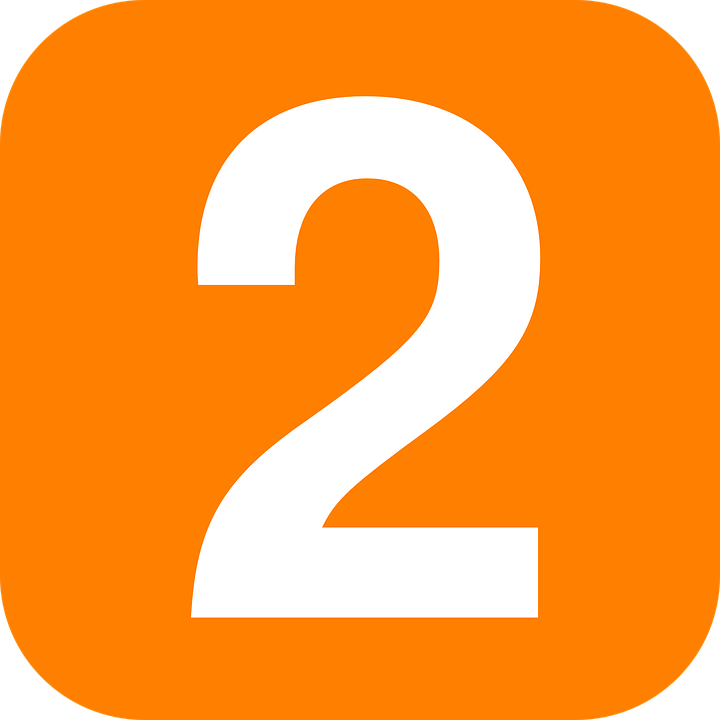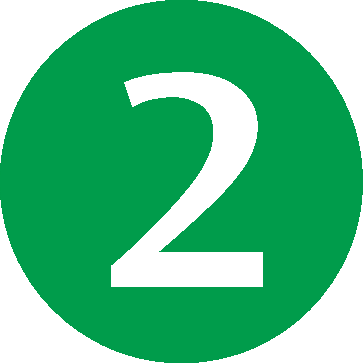There are more number 2's here.